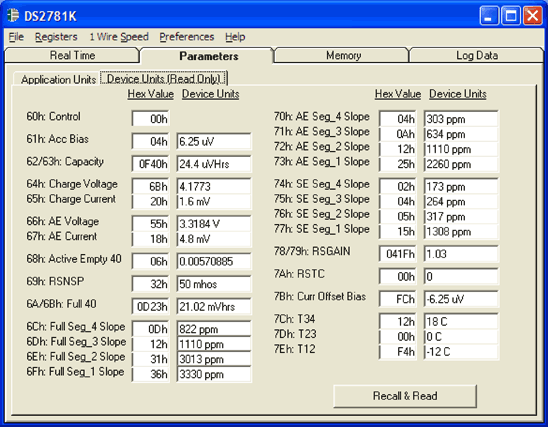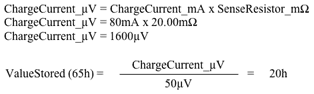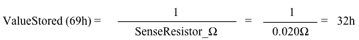# Storing Battery Fuel Gauge Parameters in the DS2781

### 要約

The DS2781 stand-alone fuel gauge allows the user to customize a stand-alone battery fuel gauge to the exact requirements of the application and cell being used. The parameters required are typically known in units such as mA, V, mAhrs, and mΩ. However, the DS2781 requires that the parameters are stored in units such as µV, µVhrs and mhos. This application note describes the calculations for converting the typical units into units that are stored in the DS2781.

### Introduction

The DS2781 two-cell, stand-alone fuel gauge is simple to use and very accurate, assuming the correct parameters have been stored in the device. It is very important that the correct data for the application is stored into the device in order optimize the fuel gauge. The DS2781K (DS2781 evaluation kit) provides an easy way to program the DS2781. The user can input characterization data of the cell and other application data in units such as mA, V, mAhrs, and mΩ, as shown in Figure 1. The DS2781K then converts that data into the correct format that gets stored to the DS2781, as shown in Figure 2. Application note 3463, "Getting Started with the DS2780K", describes how to select all of the parameters that are stored in the DS2781. Alternately, this application note describes the calculations involved in storing the parameters to the device.Figure 1. Application units sub-tab of the parameters tab.Figure 2. Device units sub-tab of the parameters tab.

### Calculations

Figure 1 shows the parameters required by the DS2781 in order to operate accurately as a fuel gauge. When the Write & Copy button is clicked, the DS2781K software converts the parameters into the format that is stored in the device, as shown in Figure 2. Those values are then written and copied into the EEPROM Addresses 60h-7Eh.

The following sections show the calculations that are required to convert the application parameters into the actual values that are stored in the DS2781 at each address. The units used in the calculations are displayed such that AccBias_µV indicates the Accumulation Bias Register is displayed in terms of µV. AccBias_mA indicates that same value in terms of mA. The value that is to be programmed to each EEPROM address is shown as a hexadecimal value in the form ValueStored (EEPROM ADDRESS). Each ValueStored shown is a single byte. Example data in the following calculations come from the Figure 1 values that are plugged into the equations to illustrate computation of the values in Figure 2.

The Control Register is stored in Address 60h, and the bits are formatted as described in the DS2781 data sheet. No calculations are required.

### Accumulation Bias Register (Address 61h)

The Accumulation Bias register is used to estimate battery currents that do not flow through the sense resistor, or battery self-discharge. This is a signed register with an LSB value of 1.5625µV/RSNS. It is stored in Address 61h and has a range of -200.000µV to 198.4375µV. Assuming the Sense Resistor has a value of 20mΩ, the range is -10mA to 9.921875mA in 78.125µA steps.The Aging Capacity register stores the rated capacity used in estimating the decrease in battery capacity that occurs during normal use. It is an unsigned register with an LSB value of 6.25µVhrs/RSNS. This register is stored in Addresses 62h and 63h and has a range of 0 to 409.59375mVhrs. Assuming the Sense Resistor has a value of 20mΩ, the range is 0 to 20479.6875mAhrs in 0.3125mAhrs steps.The Charge Voltage register stores the charge voltage threshold that detects a fully charged state. This is an unsigned register with an LSB value of 39.04mV. It is stored in Address 64h and has a range of 0 to 9.9552V.### Minimum Charge Current (Address 65h)

The Minimum Charge Current register stores the charge-current threshold that detects a fully charged state. This is an unsigned register with an LSB value of 50µV. It is stored in Address 65h and has a range of 0 to 12.75mV. Assuming the Sense Resistor has a value of 20mΩ, the range is 0 to 637.5mA in 2.5mA steps.### Active Empty Voltage (Address 66h)

The Active Empty Voltage register stores the voltage threshold that detects the Active Empty point. This is an unsigned register with an LSB value of 39.04mV. It is stored in Address 66h and has a range of 0 to 9.9552V.### Active Empty Current (Address 67h)

The Active Empty Current register stores the discharge current threshold that detects the Active Empty point. This is an unsigned register with an LSB value of 200µV. It is stored in Address 67h and has a range of 0 to 51.2mV. Assuming the Sense Resistor has a value of 20mΩ, the range is 0 to 2560mA in 10mA steps.### Active Empty 40 (Address 68h)

The Active Empty 40 register stores the Active Empty point value for +40°C (refer to Figure 12 in the DS2781 data sheet). This is an unsigned register with an LSB value of parts per million (ppm) of the Full point at +40°C. It is stored in Address 68h and has a range of 0 to 24.9% of the Full point for +40°C.### Sense Resistor Prime (Address 69h)

The Sense Resistor Prime (RSNSP) register stores the value of the sense resistor for use in computing the absolute capacity results. This is an unsigned register with an LSB value of 1mhos. It is stored in Address 69h and has a range of 1mhos to 255mhos, which is 1Ω to 3.922mΩ.The Full 40 stores the Full point value for +40°C (refer to Figure 12 in the DS2781 data sheet). This is an unsigned register with an LSB value of 6.25µVhrs/RSNS. It is stored in Addresses 6Ah and 6Bh and has a range of 0 to 409.59375mVhrs/RSNS. Assuming the Sense Resistor has a value of 20mΩ, the range is 0 to 20479.6785mAhrs in 0.3125mAhrs steps.The Full point at +40°C is a stored value (Full 40), and the Full points at the other temperatures are calculated using the slope of the Full line (refer to Figure 12 in the DS2781 data sheet). The slope of the Full line between each of the programmable temperature points (T34, T23, T12) is stored as an unsigned byte in terms of ppm/°C. It is assumed that the Full point at +40°C is the highest point on the Full line. The Full line is reconstructed in one-degree increments such that the Full point at any temperature is always less than or equal to the Full point at the next higher temperature. The slope can range from 0 to 15564ppm/°C. Note: Only three temperature points are stored in the device, but a fourth point, T01, is required to calculate the slope of Full Segment 1.

The variables used in the following equations have the following meaning and format: Full Seg_4 Slope indicates the slope from the Full point at +40°C to the Full point at temperature T34. Full_40C_mAhrs signifies the Full point in mAhrs at +40°C. Full_T34_mAhrs indicates the Full point in mAhrs at temperature T34. T34 designates the temperature breakpoint in °C. The variables used in the other equations follow the same format.### Active Empty Slopes (Address 70h-73h)

The Active Empty line is reconstructed in a similar fashion as the Full line. The Active Empty point at +40°C is a stored value (Active Empty 40), and the Active Empty points at the other temperatures are calculated from the slope between each of the temperature breakpoints (T34, T23, T12). The slopes between each of the Active Empty points are stored as an unsigned byte in terms of ppm/°C. The Active Empty line is reconstructed in one-degree increments in such a way that the Active Empty point at any temperature is always greater than or equal to the Active Empty point at the next higher temperature. The slope can range from 0 to 15564ppm/°C. Note: Only three temperature points are stored in the device, but a fourth point, T01, is required to calculate the slope of AE Segment 1.

The variables used in the following equations have the following format and meaning: AE Seg_4 Slope indicates the slope from the Active Empty point at +40°C to the Active Empty point at temperature T34. AE_40C_mAhrs shows the Active Empty point in mAhrs at +40°C. AE_T34_mAhrs denotes the Active Empty point in mAhrs at temperature breakpoint T34. T34 indicates the temperature breakpoint in °C.### Standby Empty Slopes (Address 74h-77h)

The Standby Empty line is reconstructed in a similar fashion to the Full and Active Empty lines. The Standby Empty point at +40°C is fixed at 0mAh. The Standby Empty points at the other temperatures are calculated from the slope between each of the temperature breakpoints (T34, T23, and T12). The slopes between each of the Standby Empty points are stored as an unsigned byte in terms of ppm/°C. The Standby Empty line reconstructed in one-degree increments in such a way that the Standby Empty point at any temperature the is always greater than or equal to the Standby Empty point at the next higher temperature. The slope can range from 0 to 15564ppm/°C. Note: Only three temperature points are stored in the device, but a fourth point, T01, is required to calculate the slope of SE Segment 1.

The variables used in the following equations have the following format and meaning: SE Seg_4 Slope indicates the slope from the Standby Empty point at +40°C (which is 0mAhrs) to the Standby Empty point at temperature T34. SE_40C_mAhrs signifies the Standby Empty point in mAhrs at +40°C. SE_T34_mAhrs shows the Standby Empty point in mAhrs at temperature T34. T34 indicates the temperature breakpoint in °C.### Sense Resistor Gain (Address 78h-79h)

The Sense Resistor Gain (RSGAIN) register stores the calibration factor used to produce accurate readings in the Current Register with a reference voltage forced across SNS and VSS. This register is an 11-bit value with an LSB value of 1/1024. It is stored in Addresses 78h and 79h and has a range of 0 to 1.999. The nominal value for this register is 1.000.### Sense Resistor Temperature Coefficient (Address 7Ah)

The Sense Resistor Temperature Coefficient (RSTCO) register stores the temperature coefficient of the sense resistor. This is an 8-bit value with an LSB value of 30.5176ppm/°C. It is stored in Address 7Ah and has a range of 0 to 7782ppm/°C. A value of 0 disables the temperature compensation function.### Current Offset Bias Register (Address 7Bh)

The Current Offset Bias register allows a programmable offset value to be added to raw current measurements. This is a signed register with an LSB value of 1.5625µV/RSNS. It is stored in Address 7Bh and has a range of -200.000µV to 198.4375µV. Assuming the Sense Resistor has a value of 20mΩ, the range is -10mA to 9.921875mA in 78.125µA steps.